This module defines mask transforms for weight generation.

Class labels: pixel annotations of classes (e.g., 0 for background and 1...n for positive classes)
Instance labels: pixel annotation of belonggig to different instance (e.g., 0 for background, 1 for first ROI, 2 for second ROI, etc.).

show(mask, inst_labels)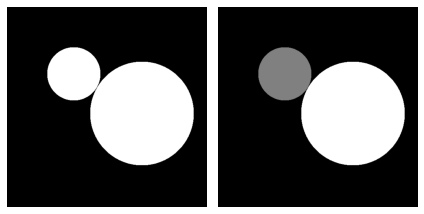The provided segmentation masks are preprocessed to

• convert instance labels to class labels
• draw small ridges between touching instances (optional)

#### preprocess_mask[source]

preprocess_mask(clabels=None, instlabels=None, ignore=None, remove_overlap=True, n_dims=2, fbr=0.1)

Calculates the weights from the given mask (classlabels clabels or instlabels).

Arguments in preprocess_masks:

• clabels: class labels (segmentation mask),
• instlabels: instance labels (segmentation mask),
• n_dims (int) = number of classes for clabels
tst1 = preprocess_mask(mask, remove_overlap=False)
show(tst1,tst2)
ind = (slice(200,230), slice(230,260))
print('Zoom in on borders:')
show(tst1[ind], tst2[ind])Zoom in on borders: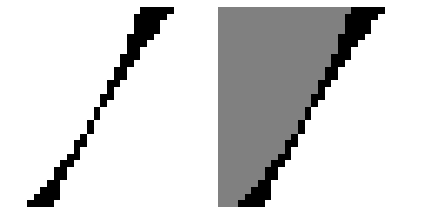Effective sampling: Probability density function (PDF)

#### create_pdf[source]

create_pdf(labels, ignore=None, fbr=0.1, scale=512)

Creates a cumulated probability density function (PDF) for weighted sampling

• labels: preprocessed class labels (segmentation mask)
• ignore: ignored reagions,
• fbr (float): foreground_background_ratio to define the sampling PDF
• scale (bool): limit size of pdf
pdf = create_pdf(tst2, scale=None)
test_eq(pdf.shape,tst2.shape*tst2.shape)
scale = 512
pdf = create_pdf(tst2, scale=scale)
test_close(pdf.max(),1)


Random center

#### random_center[source]

random_center(pdf, orig_shape, scale=512)

Sample random center using PDF

centers = [random_center(pdf, mask.shape) for _ in range(int(5e+2))]
xs = [x for x in centers]
ys = [x for x in centers]
plt.scatter(x=xs, y=ys, c='r', s=10)
plt.show()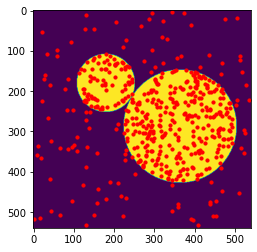We calculate the weight for the weighted softmax cross entropy loss from the given mask (classlabels).

!! Attention: calculate_weights is not used for training anymore!! See real-time weight calculation

#### calculate_weights[source]

calculate_weights(clabels=None, instlabels=None, ignore=None, n_dims=2, bws=10, fds=10, bwf=10, fbr=0.1)

Calculates the weights from the given mask (classlabels clabels or instlabels).

Arguments in calculate_weights:

• clabels: class labels (segmentation mask),
• instlabels: instance labels (segmentation mask),
• ignore: ignored reagions,
• n_dims (int) = number of classes for clabels
• bws (float): border_weight_sigma in pixel
• fds (float): foreground_dist_sigma in pixel
• bwf (float): border_weight_factor
• fbr (float): foreground_background_ratio
labels, weights, _ =  calculate_weights(clabels=mask)
titles = ['Labels (Mask)', 'Weights', 'PDF', ]
show(labels, weights)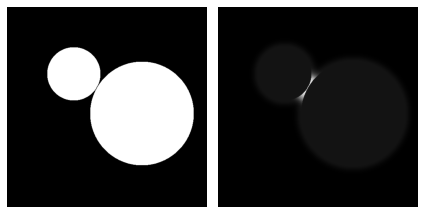Plot different weight parameters (foreground_dist_sigma_px, border_weight_factor)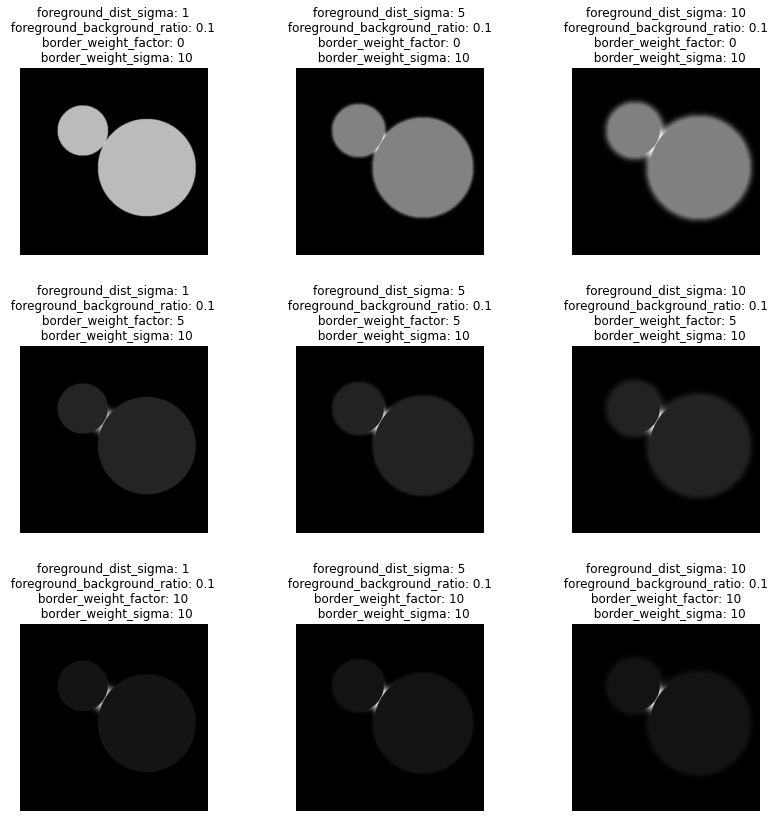### Real-time weight calculation

To efficiently calculate the mask weights for training we leverage the LogConv apporach for fast convolutional distance transform based on this paper: Karam, Christina, Kenjiro Sugimoto, and Keigo Hirakawa. "Fast convolutional distance transform." IEEE Signal Processing Letters 26.6 (2019): 853-857.

Our implementation in Pytorch leverages

• Separable convolutions
• GPU accelaration

We use a lambda=0.35 and a kernel size of 73.

#### lambda_kernel[source]

lambda_kernel(ks, lmbda)

test_eq(lambda_kernel(3, 0.35),1)


## classSeparableConv2D[source]

SeparableConv2D(lmbda, channels, ks=73, padding_mode='constant') :: Module

Apply kernel on a 2d Tensor as a sequence of 1-D convolution filters.

inp1 = torch.eye(3)[inst_labels][...,1:]
inp1 = inp1.permute(2,0,1)
tst = SeparableConv2D(0.35, channels=inp1.size(-1))
out = tst(inp1)
show(out, out)Single item version for CPU from input shape [ROIS, H, W]

## classWeightTransformSingle[source]

WeightTransformSingle(channels, bws=10, fds=10, bwf=1, fbr=0.1, lmbda=0.35, ks=73) :: DisplayedTransform

A transform with a __repr__ that shows its attrs

tst = WeightTransformSingle(channels=inp1.size(-1))
out = tst(inp1)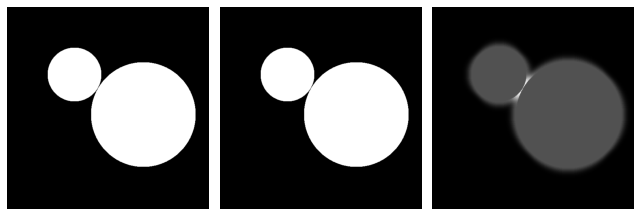Batch version for GPU transforms from instance labels with shape [batch, H, W]

## classWeightTransform[source]

WeightTransform(*args, **kwargs) :: WeightTransformSingle

A transform with a __repr__ that shows its attrs

inp2 = torch.Tensor(inst_labels)#.cuda()
inp2 = inp2.view(1, *inp2.shape)
tst = WeightTransform(channels=inp2.size(-1))
out = tst(inp2)